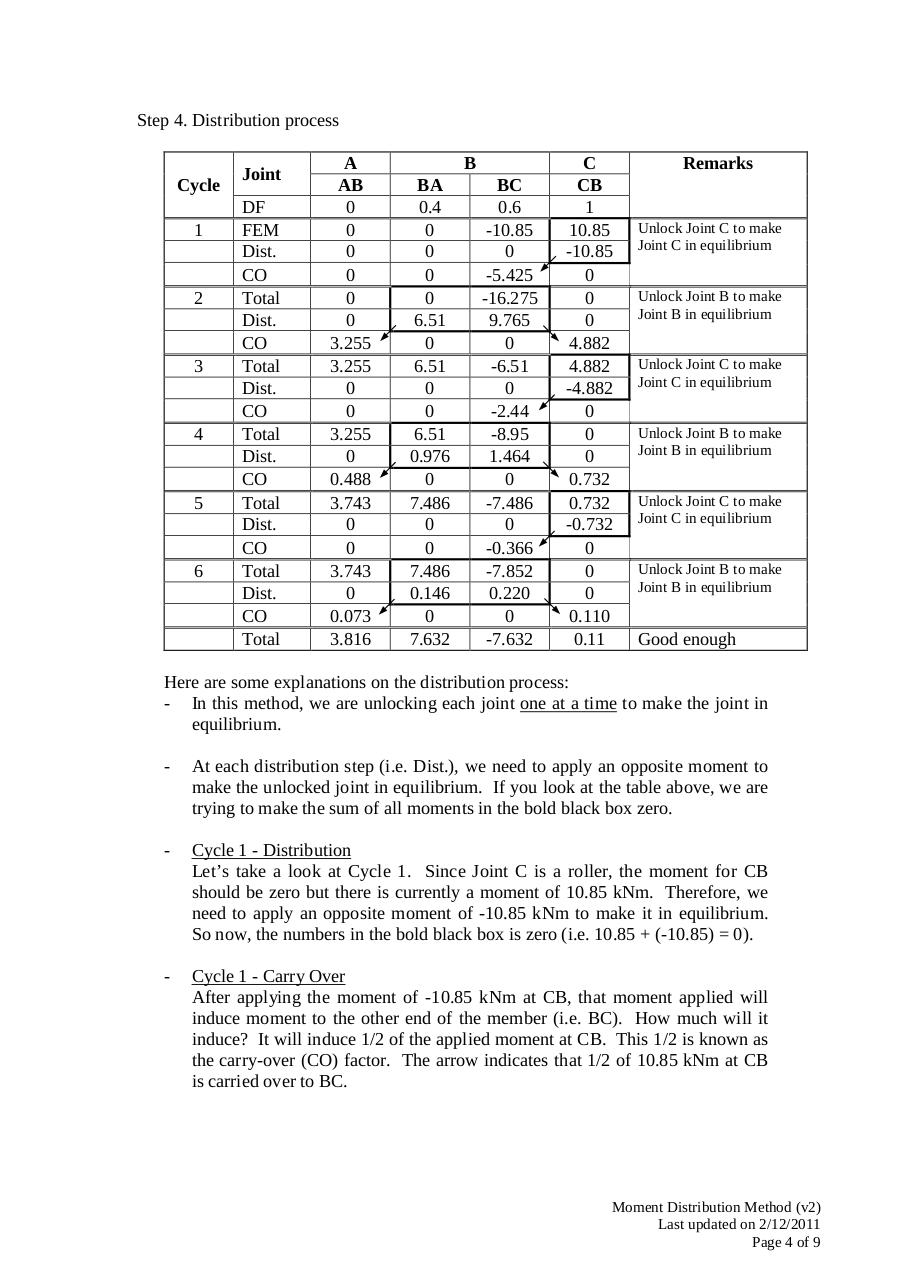# TDII MDM (v2).pdfPage 1 2 3 4 5 6 7 8 9

#### Text preview

Step 4. Distribution process

Cycle
1

2

3

4

5

6

Joint
DF
FEM
Dist.
CO
Total
Dist.
CO
Total
Dist.
CO
Total
Dist.
CO
Total
Dist.
CO
Total
Dist.
CO
Total

A
AB
0
0
0
0
0
0
3.255
3.255
0
0
3.255
0
0.488
3.743
0
0
3.743
0
0.073
3.816

B
BA
0.4
0
0
0
0
6.51
0
6.51
0
0
6.51
0.976
0
7.486
0
0
7.486
0.146
0
7.632

BC
0.6
-10.85
0
-5.425
-16.275
9.765
0
-6.51
0
-2.44
-8.95
1.464
0
-7.486
0
-0.366
-7.852
0.220
0
-7.632

C
CB
1
10.85
-10.85
0
0
0
4.882
4.882
-4.882
0
0
0
0.732
0.732
-0.732
0
0
0
0.110
0.11

Remarks

Unlock Joint C to make
Joint C in equilibrium
Unlock Joint B to make
Joint B in equilibrium
Unlock Joint C to make
Joint C in equilibrium
Unlock Joint B to make
Joint B in equilibrium
Unlock Joint C to make
Joint C in equilibrium
Unlock Joint B to make
Joint B in equilibrium

Good enough

Here are some explanations on the distribution process:
- In this method, we are unlocking each joint one at a time to make the joint in
equilibrium.
-

At each distribution step (i.e. Dist.), we need to apply an opposite moment to
make the unlocked joint in equilibrium. If you look at the table above, we are
trying to make the sum of all moments in the bold black box zero.

-

Cycle 1 - Distribution
Let’s take a look at Cycle 1. Since Joint C is a roller, the moment for CB
should be zero but there is currently a moment of 10.85 kNm. Therefore, we
need to apply an opposite moment of -10.85 kNm to make it in equilibrium.
So now, the numbers in the bold black box is zero (i.e. 10.85 + (-10.85) = 0).

-

Cycle 1 - Carry Over
After applying the moment of -10.85 kNm at CB, that moment applied will
induce moment to the other end of the member (i.e. BC). How much will it
induce? It will induce 1/2 of the applied moment at CB. This 1/2 is known as
the carry-over (CO) factor. The arrow indicates that 1/2 of 10.85 kNm at CB
is carried over to BC.

Moment Distribution Method (v2)
Last updated on 2/12/2011
Page 4 of 9